# 1st PUC Chemistry Question Bank Chapter 9 Hydrogen

You can Download Chapter 9 Hydrogen Questions and Answers, Notes, 1st PUC Chemistry Question Bank with Answers Karnataka State Board Solutions help you to revise complete Syllabus and score more marks in your examinations.

## Karnataka 1st PUC Chemistry Question Bank Chapter 9 Hydrogen

### 1st PUC Chemistry Hydrogen One Mark Questions and Answers

Question 1.
What is the strength of
(a) 10 volume H2O2
(b) 20 volume H2O2?
(a) 3%
(b) 30%.

Question 2.
Name the most abundant element in the universe.
Hydrogen.

Question 3.
Which isotope of hydrogen is radioactive?
Tritium (31H)  is radioactive.

Question 4.
Name the isotope of hydrogen which used in nuclear reactor.
21H (deuterium)

Question 5.
What is meant by hard water?
Hard water is water containing bicarbonates, sulphates and chlorides of Ca2+ and Mg2+.Question 6.
Why does elemental hydrogen react with other substances slowly at room temperature ?
It is because it has high bond dissociation energy (436 kJ mol-1)

Question 7.
What is the importance of heavy water with regard to nuclear power generation ?
It is used as coolant and moderator i.e., to slow down the fast moving neutrons.

Question 8.
Why does water has a high boiling point and a high melting point as compared to H2S?
Water molecules are associated with intermolecular H-bonding whereas H2S is not.

Question 9.
When sodium hybride is electrolysed, hydrogen is liberated at which electrode? Give equation.
At anode;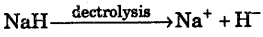at anode 2H – 2e → H2(g)

Question 10.
Which gas is evolved when Mg3N2 (Magnesium nitride) is treated with H2O? Give chemical reaction.
NH3 gas is evolved. Mg3N2 +6H2O → 3Mg(OH)2 +2NH3

Question 11.
How is heavy water produced from ordinary water?
It is obtained by repeated electrolysis of ordinary water.

Question 12.
What are the ways in which water molecules are bonded to an anhydrous salt to form a hydrate?
Co-ordinate bond and H-bond

Question 13.
Why is H2 more reactive than D2?
H – H has less bond dissociation energy than D-D

Question 14.
Which of the substances present in water cause permanent hardness of water?
CaSO4, MgSO4, CaCl2 and MgCl2 cause permanent hardness of water.

Question 15.
Permanent hardness cannot be removed by:
(a) Adding washing soda to water,
(b) Boiling water,
(c) Adding sodium polymetaphosphate to water,
(d) Passing water through ion exchange resins.
(b) Boiling water

Question 16.
How is pure H2 obtained ?
Pure H2 and O2 are obtained by electrolysis of acidified H2O.

Question 17.
What is source of sun’s energy ?
Nuclear fusion reaction.

Question 18.
Which type of bond is presents in water molecule?
Covalent bond and inter molecular Hydrogen bond.

Question 19.
Which type of oxide is water?
H2O is an amphoteric oxide.

Question 20.
Which isotope of hydrogen does not have neutron?
11H does not have neutron. It is called protium or ordinary hydrogen.Question 21.
Which out of nascent hydrogen and dihydrogen is more reactive?
Nascent hydrogen (newly bom hydrogen) [H] is more reactive than molecular hydrogen.

Question 22.
What is molar mass of heavy water?
Molar mass of heavy water is 20 g mol-1

Question 23.
How does CaC2 react with heavy water?
CaC2+2D2O → Ca(OD)2 calcium deuteroxide + C2D2 Deuteroethyne

Question 24.
Name two compounds which retard decomposition of H2O2.
Glycerol and acetanilide retard decomposition of H2O2.

Question 25.
Give two examples of interstitial hydrides.
CuH, FeH are examples of interstitial hydrides.

Question 26.
What is the tradename of sodium hexa-metaphosphate?
Calgon.

Question 27.
In a reaction of F2 and H2O, what is the role of water?
2F2+2H2O → 4HF + O2 water is acting as reducing agent.

Question 28.
What is the nature of H2O2 ?
H2O2 is acidic in nature.

Question 29.
Does hydrogen peroxide act as

• Strong acid,
• Bleaching agent ?
• No, Weak acid,
• Yes

Question 30.
Old paintings of lead are generally washed with dilute solution of hydrogen peroxide in order to regain its colour. Why?
Black colour in paintings is due to lead sulphide (PbS). This is oxidized to lead sulphate by hydrogen peroxide.

Question 31.
Melting point, enthalpy of vapourisation and viscosity data of H2O and D2O is given below: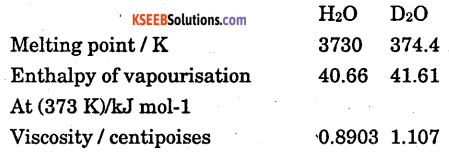On the basis of this data explain in which of these liquid intermolecular forces are stronger ?
Intermolecular forces of attraction are stronger in D2O than in water.

Question 32.
Write the Lewis structure of hydrogen peroxide.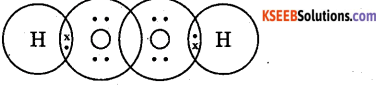Question 33.
Why is water molecule polar ?
Due to difference in electronegativity between O and H and angular shape

Question 34.
Write redox reaction between fluorine and water.
2F2(g) + 2H2O(l) → 4H(aq) + 4F(aq) + O2

Question 35.
Concentrated sulphuric acid cannot be used drying H2. Why?
Cone. H2SO4 on absorbing water from moist H2 produces so much heat that H2 catches fire.Question 36.
Why is dihydrogen gas not preferred in balloons ?
H2 is combustible in nature. Therefore it may react with oxygen violently. Thus it is not used in balloons.

Question 37.
Give one method of preparing deuterium.
It is prepared by electrolysis of heavy water (D2O) 2D2O → 2D2 + O2

Question 38.
Which salts present in water mark it permanent hard?
Calcium and Magnesium chlorides and sulphates.

Question 39.
Name a process which can remove both temporary and permanent hardness of water.
Permutite.

Question 40.
Complete the reaction : Fe(s) + H2O(g) →
3Fe(s) + 4H2O(g) → Fe3O4(s) + 4H2(g)

Question 41.
What is water gas and how it is obtained?
Water gas is mixture of CO and H2. It is obtained by passing super heated steam over hot coke.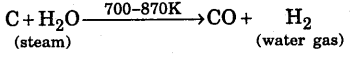Question 42.
A sample of hard water is allowed to pass through an anion exchanger. Will it produce lather with soap easily?
No. Ca2+ and Mg2+ ions are still present and these will react with soap to form curdy white ppt. Therefore it will not produce lather with soap solution easily.

Question 43.
Anhydrous BaO2 is not used for preparing H2O2. Why ?
BaSO4 formed dining the reaction of BaO2 with H2SO4 forms a protective layer around unreacted BaO2 and the reaction stops after sometime.

Question 44.
Find the volume strength of 2N H2O2 solution.
Volume strength = 5.6 × Normality
Volume strength = 5.6 × 2
= 11.2 volumes

Question 45.
Give an example each of an ionic hydride and a covalent hydride.
Ionic : NaH or CaH2
Covalent: H2O,B2H6,CH4 etc.

Question 46.
Can distilled water be called as deionised water ?
Yes, distilled water does not contain any cations and anions and hence can be called as deionized water.

Question 47.
Explain why oxide ion is called a hard ion?
Oxide ion is very small in size and thus cannot be easily polarized and hence it is called a hard ion.

Question 48.
Anhydrous BaO2 is not used for preparing H2O2. Why?
Anhydrous BaO2 is not used because the BaSO4 formed during the reaction forms a protective layer around unreacted BaO2 and the reaction stops after some time.

Question 49.
How is D2O2 prepared ?
D2O2 is prepared by distillation of potassium per sulphate (K2S2O8) with D2O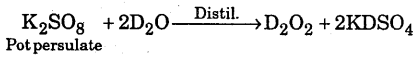Question 50.
What is hydride gap ?
The region of the periodic table from groups 7-9 which do not form hydrides is called the hydride gap.Question 51.
What is perhydrol ?
Perhydrol is the trade name for H2O2 which is used as an antiseptic for washing wounds, teeth and ears.

Question 52.
What is syngas ? Why is it called so ?
Mixtures of CO and H2 are called syngas. It is so named because it is used for the synthesis of methanol and a number of hydrocarbons.

Question 53.
What you mean by degree of hardness ?
Degree of hardness is defined as the number of parts of calcium carbonate or equivalent to various calcium and magnesium salts present in a million parts of water by mass. It is expressed in ppm.

Question 54.
For which work H.C. Very get Noble prize in 1934.
Heavy hydrogen or deuterium was separated from liquid hydrogen by fractional evaporation by H.C. Urey. For this great contribution, he was awarded the Noble Prize in Chemistry in 1934.

### 1st PUC Chemistry Hydrogen Two Marks Questions and Answers

Question 1.
Write one chemical reactions for the preparation of D2O2.
D2O2 can be prepared by the reaction of D2SO4 dissolved in water over BaO2.
BaO2 + D2SO4 → BaSO4 + D2O2

Question 2.
H2O2 is a better oxidizing agent than water. Explain.
H2O2 is better oxidising agent than water because
(a) It oxidizes an acidified KI solution to I2 which gives blue colour with starch solution but water does not.
(b) H2O2 turns black PbS to white PbSO4 but water does not.

Question 3.
Dihydrogen reacts with dioxygen (O2) to form water. Write the name and formula of the product when the isotope of hydrogen which has one proton and one neutron in its nucleus is treated with oxygen? Will the reactivity of both the isotope be the same towards oxygen? Justify your answer.
The isotope of hydrogen containing one proton and one neutron is deuterium (D).
2D2(g) + O2(g) → 2D2O Deuterium oxide
Since D-D bond is stronger than H – H bond, therefore, D2 is less reactive towards oxygen than H2.

Question 4.
Rohan heard that instructions were given to the laboratory attendant to store a particular chemical i.e., keep it in the dark room, add some urea in it, and keep it away from dust. This chemical acts as an oxidizing as well as a reducig agent in both acidic and alkaline media. This chemical is important for use in the pollution control treatment of domestic and industrial effluents.

• Write the name of this compound.
• Explain why such precautions are taken for storing this chemical?

• Hydrogen peroxide, H2O2.
• H2O2 decomposes in the presence of light and dust particles. Therefore, it is stored in wax-lined glass or plastic vessels in the presence of stabilizers like urea.

Question 5.
Why is the ionization enthalpy of hydrogen higher than that of sodium?
The size of hydrogen is smaller than that of sodium and therefore, the ionization enthalpy of H is higher (1312 kJ mol-1) than that of Na (496 kJ mol-1).

Question 6.
Basic principle of hydrogen economy is transportation and storage of energy in the form of liquid or gaseous hydrogen. Which property of hydrogen may be useful for this purpose?
Basic property of hydrogen economy is that it can be converted into liquid by cooling under high pressure and therefore, can be transported.

Question 7.
Why can dilute solution of hydrogen peroxide not be concentrated by heating?
H2O2 cannot be concentrated simply by heating because it decomposes much below its boiling point. Therefore, concentration of H2O2 is carried out in a number of stages.

Question 8.
Why is hydrogen peroxide stored in wax-lined bottles?
Hydrogen peroxide is decomposed by the rough surfaces of glass, alkali oxides present in it and light. Therefore, to prevent its decomposition, H2O2 is usually stored in coloured paraffin wax coated plastic or Teflon bottles.

Question 9.
Phosphoric acid is preferred over sulphuric acid in preparing hydrogen peroxide from peroxides. Why ?
H3SO4 acts as a catalyst for decomposition of H2O2. Therefore, some weaker acid such as H2PO4 is preferred over H2SO4 for preparing H2O2 from peroxide.

Question 10.
Atomic hydrogen combines with almost all elements but molecular hydrogen does not. Explain.
Atomic hydrogen is highly unstable and hence very reactive. Therefore, it combines with almost all the elements, However, molecular hydrogen has large bond dissociation enthalpy (458.5 kJ mol,m-1) and therefore, less reactive.Question 11.
A coulourless liquid ‘A’ contains H and O elements only. It decomposes slowly on exposure to light. It is stabilized by mixing urea to store in the
presence of light,
(i) Suggest possible structure of A.
(ii) Write chemical equations for its decomposition reaction in light.
The liquid A is hydrogen pexoxide (H2O2)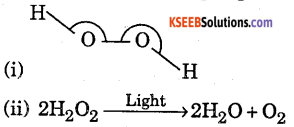Question 12.
An ionic hydride of an alkali metal has significant covalent character and is almost unreactive towards oxygen and chlorine. This is used in the synthesis of other useful hydrides. Write the formula of this hydride. Write its reaction with Al2Cl6.
Since the ionic hydride of alkali metal has significant covalent character, therefore, it is LiH. Since LiH is very stable, therefore, it is almost unreactive towards O2 and Cl2. It reacts with Al2Cl2 form lithium aluminium hydride.
8LiH + Al2Cl6 → 2LiAlH4+6LiCl

Question 13.
Hydrogen is not prepared by the reaction of concentrated sulphuric acid on zinc. Explain.
Concentrated sulphuric acid is not used for the preparation of hydrogen because it reacts with zinc to form SO2 gas instead of hydrogen.
Zn + 2H2SO4 → ZnSO4 + 2H2O + SO2

Question 14.
Which element can oxidize water to O2?
Fluorine being more electronegative than oxygen can oxidize water to oxygen. In this, water acts a reducing agent and hence itself gets oxidized to O2.
2F2(g) + 2H2O(l) → 4H+(aq) + 4Fm(aq) + O2(g)

Question 15.
Dilute solution of hydrogen peroxide cannot be heated strongly for its concentration. Explain.
Dilute solution of H2O2 cannot be concentrated by heating because it decomposes into H2 and O2 on heating.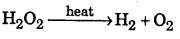Question 16.
Can sodium bicarbonate make water hard?
Sodium bicarbonate cannot make water hard because soaps themselves are sodium salts of fatty acids which are soluble in water.

Question 17.
Which of the two hydrogen or deuterium undergoes reactions more rapidly and why?
Hydrogen undergoes reactions more rapidly than deuterium. This is because of mass differences. For example, reaction between hydrogen and chlorine is about 14 times faster than between deuterium and chlorine.

Question 18.
Can we remove completely temporary hardness due to Mg(HCO3)2 by boiling?
Temporary hardness of water due to Mg(HCO3)2 can be completely removed by boiling because soluble Mg(HCO3)2 is converted into insoluble MgCO3 which can be easily removed by filtration.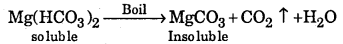Question 19.
H2O2 acts as an oxidizing agent as well as reducing agent. Why ?
In H2O2 , oxygen has -1 oxidation state which lies between maximum (0 or +2 in OF2) . and minimum -2. Therefore, oxygen can be oxidized to O2 (zero oxidation state) acting as reducing agent or can be reduced to H2O or OH- ( -2 oxidation state) acting as an oxidizing agent.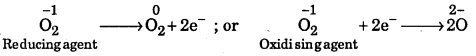Question 20.
Why do lakes freeze from top towards bottom ?
The upper surface of lake is in contact with the cold environment and starts to freeze. The density of ice is less than that of liquid water. Therefore, ice floats on the surface. Thus, the ice layer at lower temperature floats over the water below it. Due to this the freezing of water into ice occurs continuously from top towards bottom.Question 21.
A mixture of H2O2 and hydrazine with copper (II) is used as a rocket propellant. Why ?
The reaction between hydrazine (NH2NH2)and H2O2 in the presence of Cu(II) is highly exothermic and is accompanied by a large increase in energy as well as in volume of the products. Therefore, this mixture is used as a rocket propellant.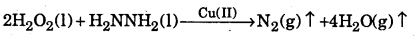Question 22.
Water cannot be used to extinguish petrol fires. Why?
Water is used to extinguish most fires because it lowers the temperature of burning material. However, in case of petrol fires, petrol being lighter than water, floats over water and hence fire spreads instead of being extinguished.

Question 23.
Ferric chloride is reduced when zinc and hydrochloric acid are added to its solution and not by passing H2 gas through its solution. Explain.
Ordinary H2 is less reactive and therefore, it does not reduce acidified FeCl3 solution. However, when zinc is added to acidified FeCl3 solution, nascent hydrogen is produced which has enormous energy. It is more reactive than ordinary H2 and reduces acidified FeCl3 solution.
FeCl3 + H2 → No reaction; Zn + H2SO4 → ZnSO4 + H2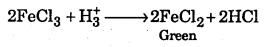Question 24.
Hard water is softened before using in boilers. Explain
Hard water on boiling forms precipitates of MgCO3, CaCO3 and CaSO4 which form scales in the boilers. As a result of these scales in the boilers. The boiler gets deteriorated due to over heating. Moreover, these scales are non-conducting and therefore, more fuel is consumed. Therefore, in order to prevent the formation of scales, hard water softened before using in boilers.

Question 25.
What are the uses of Heavy Water?
(a) Heavy water is used as a coolant and moderator for neutrons in nuclear reactors.
(b) Heavy water is used in the preparation of deuterium and deuterated compounds.
(c) It is used as a tracer element in metabolic processes.

Question 26.
Presence of water is avoided during the preparation of H2O2 from Na2O2 Why?
Water present during the reaction, reacts with Na2O2 form NaOH which tends to decompose H2O2.
Na2O2 + 2H2O → H2O2 +2NaOH
2H2O2 → 2H2O + O2

Question 27.
Discuss the position of hydrogen in the periodic table is not justified.
Its position in periodic table is not justified because it resembles both alkali metals and halogens.

• Its electronic configuration is similar to alkali metals.
• It is non-metal like halogens.
• Its ionization energy is high like halogens.
• It can lose electrons to form H+ like alkali metals.

Question 28.
Distinguish clearly between salt-like and covalent hydrides.
Salt-like hydrides are also called ionic hydrides. They are formed by group 1 and 2 elements with H2. They are basic in nature. They dissolve in water forming alkali and give H2 gas, .e.g., NaH, CaH2, KH etc.
Covalent hydrides are formed between less electropositive or electronegative elements.
Ex : B2H6, AlH3, BeH2, They are insoluble in water.

Question 29.
Describe the industrial use of hydrogen which depends on
(a) the heat liberated when it burns
(b) its ability to react with vegetable oil in the presence of a catalyst
(c) its ability to unite with N2.
(a) It is used in oxy-hydrogen flame for welding purpose.
(b) It is used to manufacture vanaspati ghee.
(c) It is used for manufacture of NH3.

Question 30.
(a) How would you prepare dihydrogen from water by using a reducing agent?
(b) How would you prepare dihydrogen from a substance other than water?
(c) How would you prepare very pure H2 in the laboratory?
(d) How would you prepare heavy hydrogen in the laboratory?
(a) 2Na + 2H2O → 2NaOH + H2
(b) Zn + 2HCl → ZnCl2 + H2
(c) It is obtained by electrolysis of acidified water.
(d) It is obtained by electrolysis of heavy water.Question 31.
What are the products of the following reactions ? Write balanced equation
(i) FeO(s) + H2(g) →
(ii) MnO4 + H2O2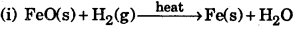(ii) 6H+ + 2MnO4 + 5H2O2 > 2Mn2+ + 8H2O + SO2

Question 32.
State whether each of the following compounds is a acidic anhydride. Write a balanced equation for the reaction with water in each : Na2O, P4O6 SO2, Al2O3
Na2O is base, Na2O + H2O → 2NaOH
P4O6 is basic, P4O6 + 6H2O →4H3PO3AlO3 is amphoteric oxide (acidic as well as basic)

Question 33.
Describe the structure of common form of ice.
In the normal hexagonal ice, each oxygen atom is tetrahedrally surrounded by four other oxygen atoms, there being an hydrogen atom in between each pair of oxygen. Each hydrogen is covalent bonded to one oxygen and linked to the other oxygen by a covalent bond. Such an arrangement leads to packing having large open spaces. The density of ice is therefore less than liquid water.Question 34.
Distinguish clearly between (a) hard and soft water, (b) temporary hardness and permanent hardness.

 Hard Water Soft Water 1. water which contains soluble of Ca2+, Mg2+. 1. It does not contain Ca2+ and Mg2+. 2. It does not form lather with soap easily. It forms precipate of insoluble substances with soap 2. It forms lather with soap.
 Temporary hardness Permanent hardness 1. It is caused by bicarbonates of Ca2+ and Mg2+. 1. It is due to chlorides and sulphates of Ca2+ and Mg2+. 2. It is removed by boiling. 2. It is removed by heating with washing soda.

Question 35.
How is H2O2 manufactured ?
Hydrogen peroxide is manufactured by electrolysis of 50% H2SO4.Question 36.
What is the structure of H2O2? Draw a schematic diagram indicating the shape of the molecule clearly.
Hydrogen peroxide has open-book structure. It has non-planar structure. In the crystal, the dihedral angle 111.5° reduces to 90.2° on account of hydrogen bonding. There is single bond between two oxygen atom in hydrogen peroxide.Question 37.
Show how H2O2 functions as a as an oxidizing agent with two examples.
Oxidising agent:

• PbS + 4H2O2 → PbSO4 + 4H2O
• 21 + H2O2 + 2H → I2+2H2O

Question 38.
Show how H2O2 functions as oxidising agent with two examples.
Oxidising agent: PbO2 + H2O2 → PbO + H2O + O2
H2O2 + Ag2O →H2O + O2 + 2Ag

Question 39.
H2O2 is used to restore the colour of old paintings containing lead sulphide. Write a balanced equation for the reaction that takes place in this process.Question 40.
What is the mass of H2O2 present in 1 litre of a 2M solution ? Calculate the volume of oxygen at STP liberated upon the complete decomposition of 100 cm3 of the above solution.
1 litre of 1M solution of H2O2 contains 34g of H2O2 [(2H = 2) + (20 = 32) = 34]
I litre of 2 M solution of H2O2 contains 34 × 2 = 68g of H2O2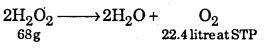1000 ml of 2M solution contains 68g of H2O2
100 ml of 2M solution contains 6.8g of H2O2
68g of H2O2 will produce 22.4 litre of O2 at STP
6.8 g of H2O2 will produce = 2.24 litre of O2 to STP.Question 41.
Ionisation energy of hydrogen is much higher than those of alkali metals. In case of former combines with latter, which type of compound will be formed? Explain when H2 reacts with C at high temperature, what type of hydride will be formed. Give chemical equation.
Ionic hydride will be formed, e.g.,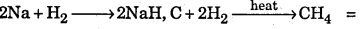Methane gas a covalent hydride is formed :

Question 42.
Fill in blanks:

1. Hydrogen shows character as well as …………………. character.
2. H+ ion in water exists ……………………. ion called ……………………….. ion.
3. Hydrogen is liberated at …………………………….. when NaH is electrolysed.
4. Hydrogen shows ………………………. and ………………………… oxidation state in compounds.

1. Electropositive, electronegative,
2. H3O+, hydronium,
3. anode,
4. +1 and – 1

Question 43.
How does H2O2 react with KMnO4 in acidic medium ? Write four uses of H2O2.
2KMnO2 + 3H2SO4 + 5H2O2 → K2SO4 + 2MnSO4 + 8H2O + 5O2
Uses :

• It is used as oxidizing agent,
• It is used as reducing agent.
• It is used as bleaching agent,
• It is used as rocket propellant.

Question 44.
Sea water can’t be used in boiler. Explain given chemical equations.
Sea water contains soluble salts of calcium and magnesium, which on boiling change to insoluble salts and get deposited as a crust on the inner sides of a boiler. This crust acts as a bad conductor of heat and prevent the flow of heat to water. A large quantity of fuel gets wasted.
Chemical Reaction
$$\begin{array}{l}{\mathrm{Ca}\left(\mathrm{HCO}_{3}\right)_{2} \stackrel{\Delta}{\longrightarrow} \mathrm{CaCO}_{3}+\mathrm{CO}_{2}+\mathrm{H}_{2} \mathrm{O}} \\ {\mathrm{Mg}\left(\mathrm{HCO}_{3}\right)_{2} \stackrel{\Delta}{\longrightarrow} \mathrm{MgCO}_{3}+\mathrm{CO}_{2}+\mathrm{H}_{2} \mathrm{O}}\end{array}$$

### 1st PUC Chemistry Hydrogen Three Marks Questions and Answers

Question 1.
Sample of hydrogen peroxide solution is 1.5 M. If it is to be labelled ‘X’ volume; what is the value of ‘X’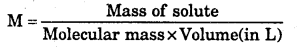Mass of H2O2 per liter = 1.5 × 34 = 51 g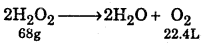68 g of H2O2 at STP gives 22.4 L of O2
51 g of H2O2 gives at STP = $$\frac{22.4 \times 51}{68}$$
= 16.8 L of H2O2 that has 51g of H2O2 will give 16.8 L of O2
Hence value of X is 16.8 L.
∴ The given sample of H2O2 is 16.8 Vol.

Question 2.
Explain with examples (i) Polymeric hybrids (ii) Complex hydrides.
Polymeric hydrides: These are formed by elements having electro negativity in the range 1.40 – 2.0. These usually exist in the polymeric form in which the monomer molecules are held together in two or three dimensions by hydrogen bridges. Some common examples are : (BeH2)n, (AlH3)n, (InH3)n , (GaH3)n , (SpH4)n
These are amorphous solids which decompose above 523 K to evolve H2 gas.
Structure. Beryllium hydride exits in the polymeric form involving three-center-two electron bonds as shown below :

Complex hydrides: In these hydrides, the hydride ion (H) acts as the ligand and is attached to the central metal atom by coordinate bonds. These are formed both by transition elements and non-transition elements. Among the non-transition elements, the most important complex hydrides are formed by elements of group 13. These are sodium borohydride (NaBH4), lithium borohydride (LiBH4) and lithium aluminium hydride (LiAlH4). These are versatile reducing agents and are widely used for reduction of organic compounds.

Question 3.
In what respects does hydrogen resemble alkali metals ? How does it resemble halogen ?
(i) Resemble with alkali metals :

• It has one valence electron in s-orbital like alkali metals
• It can lose one electron to form H+ ion like alkali metals.
• It is liberated at cathode during electrolysis of compounds like H2O, HCl etc.
• It shows +1 oxidation state like alkali metals.
• It is a strong reducing agent like other alkali metals.

(ii) Resemblance with halogen :

• Hydrogen is non-metal like halogens.
• Hydrogen forms diatomic molecule like halogens.
• It has ionization energy high like halogens.
• Its electronegativity is similar to halogens.
• It can gain one electron to form H- ion.
• When NaH is electrolysed, hydrogen is liberated at anode like halogens.Question 4.
In what respects H2 differs from alkali metals and halogens ?
Difference with alkali metals.

• Hydrogen is a non-metal whereas alkali metals are most electropositive elements.
• Hydrogen form diatomic molecules whereas alkali metals do not.
• Hydrogen mostly forms covalent compounds whereas alkali metals mostly from ionic compounds.
• The ionization energy of hydrogen is much higher than that of alkali metals.
• The size of H+ is smaller than those of alkali metals.

Difference with halogens

• It has less tendency to form hydrides (H) as compare to halogens.
• It does not have lone pair of electrons like halogens.
• It is less reactive than halogens due to high bond dissociation energy.

Question 5.
Complete the following reaction
(i) Na + H2
(ii) N2 + H2
(iii)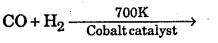(iv) $$\mathrm{H}_{2}+\mathrm{Cl}_{2} \stackrel{\text { Sunlight }}{\longrightarrow}$$
(v) H2+Br2 →
(vi) $$\mathrm{CuO}(\mathrm{s})+\mathrm{H}_{2} \stackrel{\text { heat }}{\longrightarrow}$$
(i) Na + H2 → 2NaH
(ii) N2(g) + 3H2(g) →2NH3(g)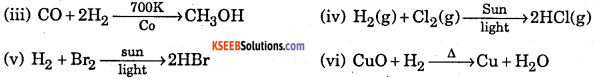### 1st PUC Chemistry Hydrogen Four Marks Questions and Answers

Question 1.
Make a comparison of atomic hydrogen with nascent hydrogen any two. How the active hydrogen and heavy hydrogen are formed ?
(a) Comparison of atomic and nascent hydrogen
Main points of differences are :

1. Nascent hydrogen can be produced even at room temperature but atomic hydrogen is produced only at very high temperature.
2. Nascent hydrogen can never be isolated, but atomic hydrogen can be isolated.
3. Reducing power of atomic hydrogen is much greater than that of nascent hydrogen.
4. Reactivity of the three forms of hydrogen increases in the order.
Molecular hydrogen (H2) < Nascent hydrogen < Atomic hydrogen.

(b) 1. Active hydrogen: It is obtained by subjecting stream of molecular hydrogen at ordinary temperature to silent electric discharge at about 30,0000 volts. It is very reactive in nature (half-life = 0.33 second) and combines directly at ordinary temperatures with Pb and S forming their hydrides.

2. Heavy hydrogen: It is manufactured by the electrolysis of heavy water containing a little of H2SO4 or NaOH to make the solution conducting.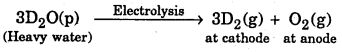In the laboratory, it can be prepared by the action of heavy water on sodium metal.
2D2O(1) + 2Na(s) → 2NaOD(aq) + D2 (g)

Question 2.
Describe the different method by which concentration of Hydrogen peroxide.
Concentration of hydrogen peroxide. Hydrogen peroxide obtained by any method is always in the form of a dilute solution. A great care is to be taken for concentrating its solution because it is unstable and decomposes on heating.
2H2O2 → 2H2O + O2
The decomposition of H2O2 is catalysed by the ions of heavy metals present as impurities. The solution of H2O2 is concentrated by following methods.
(1)By careful evaporation on a water bath. A dilute solution of H2O2 is taken in evaporation dish and is heated at 313-323 K. Water evaporates slowly and hydrogen peroxide solution of about 45-50% strength is obtained.

(2) By dehydration in a vacuum desiccators. The dilute (50%) solution of H2O2 obtained is further concentrated by placing the same in a vacuum desiccators containing concentrated H2SO4 as a dehydrating agent. Here water vapours are absorbed by concentrated sulphuric acid. This is shown in the diagram.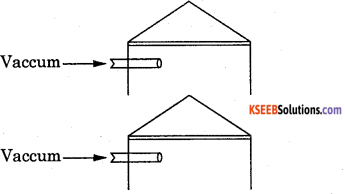(3) By distillation under reduced pressure. The solution of hydrogen peroxide is further concentrated by subjecting it to distillation under reduced pressure. The solution is distilled at 308-313 K under a reduced pressure of 15 mm Hg. Water present in the solution distills over leaving behind about 98-99% concentrated solution of hydrogen peroxide.

(4) By crystallization. The last traces of water present in H2O2 are removed by freezing it in a freezing mixture of solid CO2 and ether. The crystals of hydrogen peroxide separate out. These crystals are removed, dried and then remelted to obtained 100% pure hydrogen peroxide.

(5) Storage of hydrogen peroxide. In order to check the decomposition of hydrogen peroxide, a small amount of acetanilide (i.e., negative catalyst) is added to it before storing the hydrogen peroxide.
Hydrogen peroxide cannot be concentrated by distillation at ordinary pressure because it undergoes decomposition into water and oxygen as it is highly unstable liquid. It decomposes even on long standing or on heating.

Question 3.
What different methods are used for softening the hard water ? Explain the principle used in each method ? (Write any two)
Hard water can be softened by the following methods depending upon the nature of hardness.
(a) Temporary hardness :
1. By boiling. It can be removed by merely boiling the water. Boiling decomposes the bicarbonates to give carbon dioxide and insoluble carbonates, which can be removed by filtration.2. Clark’s process : Temporary hardness can be removed, by the addition of a calculated amount of lime, where magnesium carbonate or calcium carbonate is precipitated.
Ca(HCO3)2 + Ca(OH)2 → 2CaCO3+2H2O
Mg(HCO3)2 +Ca(OH)2 → CaCO3+MgCO3 +2H2O

(b) Permanent hardness: With sodium carbonate. On treatment with washing soda, Ca2+ and Mg2+ in hard water are precipitated. The precipitate of the insoluble carbonates thus formed is removed by filtration.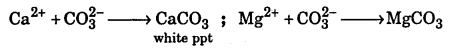The anion may remain in solution but in this form it is not dangerous.

(ii) Ion-exchange method. The common substances used for this process is zeolite which is hydrated sodium aluminium silicate, NaAl(SiO2)2. The exchange occurs when on passing over the zeolite bed, sodium ions from zeolite are replaced by calcium and magnesium ions. Thus .
2NaZe + Ca2+ → (Ze)2 Ca + 2Na+ ; 2NaZe + Mg2+ → (Ze)2 Mg + 2Na+
When all the sodium ions of zeolite has been replace, the zeolite is said to be exhausted. It can be regenerated by treatment with strong solution of sodium chloride.
2Na + (Ze)2 Ca → 2ZeNa + Ca2+

Question 4.
How can you show that H2O2 works both as oxidizing and reducing agent by giving examples.
Oxidising properties. H2O2 has a tendency to accept electrons in chemical reactions and thus behaves as an oxidizing agent in both acidic and alkaline medium.
(a) In acidic medium
H2O2 → H2O + O
H2O2 + 2H+ + 2e → 2H2O
Example:
2Fe2+ + 2H+ + H2O2 → 2Fe3+ + 2H2O
b) In alkaline medium H2O2 + OH + 2e
Example:
$$3 \mathrm{Cr}^{3+}+4 \mathrm{H}_{2} \mathrm{O}_{2}+10 \mathrm{OH}^{-} \longrightarrow 2 \mathrm{CrO}_{4}^{2-}+8 \mathrm{H}_{2} \mathrm{O}$$

Reducing properties. H2O2 can give electrons in a few reactions and thus behaves as a reducing agent.
a) In acidic medium, H2O2 → O2 + 2H+ + 2e
$$2 \mathrm{MnO}_{4}^{-}+6 \mathrm{H}^{+}+5 \mathrm{H}_{2} \mathrm{O}_{2} \longrightarrow 2 \mathrm{M}^{2+}+8 \mathrm{H}_{2} \mathrm{O}+5 \mathrm{O}_{2}$$

b) In alkaline medium, H2O2 + 2OH → 2H2O + O2 + 2e
2Fe3+ + H2O2 + 2OH → 2Fe2+ + O2 + 2H2OQuestion 5.
A colourless and odourless gas is used for the hydrogenation of vegetable oils, name the gas. Also name the process when the gas reacts with dinitrogen at 673 K, 200 atm and in presence of Fe as catalyst; to produce another gas that gives brown precipitate with Nessler’s reagent.
The agent is H2 (dihydrogen)
It is a Haber’s process. The reaction isQuestion 6.
(a) What do you understand by metallic hydrides?
(b) Give four uses of hydrogen peroxide.
(a) Metallic hydrides are hydrides formed by transition metals with hydrogen. They are non-stoichiometric compounds, i.e., they do not have fixed composition.

(b)

• It is used as oxidizing agent and reducing agent.
• Its dilute solution is used as mild antiseptic.
• It is used as bleaching agent.
• Cone. H2O2 is used as rocket fuel.

Question 7.
Write balanced equations for the following:
(a) Hydrated Barium peroxide reacts with orthphosphoric acid.
(b) H2O2 is allowed to react with PbS (lead sulphide)
(a) 3BaO2.8H2O + 2H3PO4 → Ba3 (PO4)2 + 2H2O2 + 23H2O
(b) PbS + 4H2O2 →PbSO4 +4H2O2

Question 8.
How is hydrogen peroxide prepared industrially ? Explain why it is stored in coloured wax-lined glass or plastic bottles ?
Industrially, H2O2 is prepared by the auto oxidation of 2-ethylanthraquinol to 2-ethylanthraquinone. H2O2 is stored in wax-lined glass or plastic bottles so that it does not decompose into H2O and O2 .
Because it is decomposed to H2O and O2 when comes in contact with sunlight.

Question 9.
(a) Distinguish between temporary hardness and permanent hardness.
(b) What happens when lead sulphide is reacted with hydrogen peroxide solution ?
(a)

 Temporary Hardness Permanent Hardness 1. It is caused by bicarbonates of Ca2+ and Mg2+ 1. It is caused by sulphates and chlorides of Ca2+    and Mg2+ 2. It is removed by boiling. 2. It is removed by adding washing soda.

(b) PbS04 is formed which is white PbS + 4H2O2 → PbSO4 + 4H2O

Question 10.
(a) What is the importance of heavy water with regard to nuclear power generation?
(b) What happens when acidified ferrous sulphate solution is reacted with hydrogen peroxide solution ?
(a) It is used as coolant and moderator in nuclear reactor.
(b) 2FeSO4 +H2SO4 +H2O2 → Fe2(SO4)3 +2H2O(I), have hydrogen peroxide acts as oxidizing agent.

Question 11.
Why hard water does not form lather with soap? What advantage has soap over detergents?
It is because Ca2+ and Mg2+ ions present in hard water react with soap to form Ca2+ and Mg2+ salts of fatty acids which are insoluble in water.
Advantages of soap over detergent; soap are biodegradable where as detergents are non-biodegradable.

Question 12.
Advantage of soap over detergent : Soaps are biodegradable whereas detergents are non-biodegradable.
Difference in chemical behaviour of compound of hydrogen with elements of atomic number 17 and 20.
Atomic number 17 = Chlorine, and Altomic number 20 = Calcium.
(b) H2 + Cl2 → 2HCl
Cl2 is oxidizing agent, H2 is reducing agent.
Ca + H2 → CaH2

Question 13.
Reaction of steam on hydrocarbon or coke at high temperature in presence of catalyst yields a mixture of gases.
(a) Name the gases produced.
(b) Write a reaction of the above mentioned process.
(c) What is a specific name given to the mixture of gases?
(d) Write the reaction when the mixture of gases is reacted with steam in presence of iron chromate as catalyst.
Also give the special name of this reaction.
(a) Carbon monoxide and dihydrogen(c) Synthetic gas or syngas or water gas.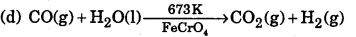Water-gas shift reaction is the special name of this reaction.Question 14.
The mixture of hydrazine and hydrogen peroxide with copper (II) catalyst is used as a rocket propellant. Why ? Write the reactions involved.
When hydrazine and hydrogen peroxide react in presence of Cu2+ Catalyst the following reaction is takes place.
$$\mathrm{N}_{2} \mathrm{H}_{4}(\mathrm{l})+2 \mathrm{H}_{2} \mathrm{O}_{2}(\mathrm{l}) \stackrel{\mathrm{Cu}^{2+}}{\longrightarrow} \mathrm{N}_{2}(\mathrm{g})+4 \mathrm{H}_{2} \mathrm{O}(\mathrm{g})$$
Since the formation of nitrogen and steam is accompanied with evolution of large amount of heat thus act as a rocket propellant.

Question 15.
A gas, A obtained by heating aluminum with sodium hydroxide was passed over a black solid, B at 773K when a grey metal, C and a colourless liquid, D were obtained. The metal C displaces copper from copper sulphate solution and becomes passive on treatment with cone. IINO3. Identity A, B, C and D and explain all the reactions.
(i) 2Al(s) + 2Na0H)(aq) + 2H2O(l) → 2NaAlO2(aq) + 3H2(g) Dihydrogen(A)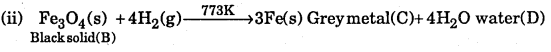(iii) Fe(s) + CuSO4(aq) → FeSO4(sq) + Cu(s)Iron is rendered passive due to the formation of ferrosoferric oxide or magnetic oxide (black solid (B))

Question 16.
Hydrogen peroxide acts both as an oxidizing agent and as a reducing agent in alkaline solution towards certain first row transition metal ions. Illustrate both these properties of H2O2 using chemical equations.
Oxidising agent: 2Cr(OH)3 + 4NaOH + 3H2O2 → 2Na2CrO4 + 8H2O
Here, Cr3+ gets oxidized to Cr6+
Reducing agent: 2K3[Fe(CN)6] + 2KOH + H2O2 → 2K4 [Fe(CN)4 ] + 2H2O + O2
Here, Fe3+ gets reduced to Fe2+.

Question 17.
A white is either Na2O or Na2O2. A piece of red litmus paper turns white when it is dipped into a freshly made aqueous solution of the white solid
1. Identify the substance and explain with balanced equation.
2. Explain what would happen to the red litmus if the white solid were the other compound.
1. Na2O2 + 2H2O → 2NaOH + H2O2
H2O2 thus produced turns red litmus paper white due to its bleaching action.

2. Na2O + H2O → 2NaOH
NaOH thus produced will turn red litmus blue.

Question 18.
The process $$\frac { 1 }{ 2 }$$ H2(g) + e → H (g) is endothermic ((AH =+151kJmor-1), yet salt like hydrides are known. How do you account for this?
It is true that formation of hydride(H) ion is an endothermic process, yet alkali and alkaline earth metals from salt like hydrides. This is due to the reason that high lattice energy released (energy released during the formation of solid metal hydrides from their corresponding ions, i.e., M+ and H) more than compensates the energy needed for the formation of H ions from H2 gas.1st PUC Chemistry Hydrogen Numerical Solved Examples:

Question 1.
Calculate the volume strength of a 3% solution of H2O2 .
100 mL of solution contains H2O2 = 3g
1000 mL of solution will contain H2O2 = $$\frac { 3 }{ 100 }$$ x lOOO = 30g
H2O2 decomposes:68g of H2O2 give at NTP = 22.4L
30g of H2O2 will give O2 at NTP = $$\frac { 22.4 }{ 68 }$$ x 30 = 9.88L = 9880mL
But 30g of H2O2 is present in 1000 mL of H2O2
1000 mL of H2O2 solution gives O2 at NTP = 9880 mL
lmL of H2O2 solution will give O2 at NTP = $$\frac { 9880 }{ 1000 }$$ = 9.88 mL
Hence volume strength of 3% H2O2 = 9.88

Question 2.
30 mL of H2O2 solution after acidification required 30 mL of N/10 KMnO4 solution for complete oxidation. Calculate the percentage and volume strength of H2O2 solution.
To calculate the normality of H2O2 solution, apply normality equation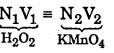For H2O2 , V1 = 40mL, N1 =.? ; For KMnO4, V2 = 30, N2 = $$\frac { N }{ 10 }$$
N1 × 30 = $$\frac { N }{ 10 }$$ x 30 N1 = $$\frac { N }{ 10 }$$ x $$\frac { 30 }{ 30 }$$ = $$\frac { 1 }{ 10 }$$ N = 0.1N
Strength = Equivalent wt x Normality = 17 × .01 = 1.7 g/L
% of strength = $$\frac { 1.7 }{ 1000 }$$ × 100 = 0.17%
H2O2 decomposes as :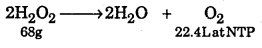68g of H2O2 give O2 at NTP = 22.4L
1.7g of H2O2 will give O2 at NTP = $$\frac { 22.4 }{ 68 }$$ × 1.7 = 0.56L

Question 3.
Calculate the normality of 20 volume solution of H2O2.
20 volume of H2O2 solution means that 1L of this solution will liberate 20L of O2 at NTP22.4L of O2 at NTP produced from H2O2 = 68g
20L of O2 at NTP is produced from H2O2 $$\frac { 68 }{ 22.4 }$$ × 20 = 60.71g
Strength of H2O2 = 60.71 g/L
Gram equivalents of H2O2 = $$\frac { 60.71 }{ 17 }$$ = 3.57
Normality = $$\frac { 3.57 }{ 1 }$$ =3.57 N

Question 4.
Calculate the amount of Hydrogen peroxide presents in 10 ml of 25 volume solution of H2O2 .22.4L of O2 at NTP is produced from H2O2 = 68g
25 L of O2 at NTP is produced from H2O2 = $$\frac { 68 }{ 22.4 }$$ × 24 = 75.9g
Strength of 25 volume H2O2 = 75.9 g/L
Amount of H2O2 present in lOmL = $$\frac{75.9}{\mathrm{H}_{2} \mathrm{O}_{2}}$$ × 10 = 0.759g

Question 5.
10 ml of given H2O2 solution contains 0.91 g of H2O2. Express its strength in volumes.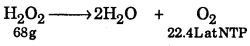Amount of H2O2 in 1L $$\frac{0.91 \times 1000}{10}$$ = 91g
68g of H2O2 give O2 at NTP = 22.4L
91 g of will give O2 at NTP = $$\frac{22.4}{68}$$ × 91 = 30
Since 91 g of is present in 1L, Volume strength = 30 volume

Question 6.
Calculate the strength of 5 volume H2O2 solution.
5 volume H2O2 solution means that 1L of 5 volume H2O2 on decomposition gives 5L of O2 at NTP.22.4 of O2 at NTP is produced from H2O2 = 68 g
5 L at O2 at NTP is produced from H2O2 = $$\frac { 68 }{ 22.4 }$$ x 5 = 15.18 g
But 5L of O2 at NTP is produced from 1L of 5 volume H2O2
Strength of H2O2 in 5 volume H2O2 = 15.18 g/L
Percentage strength of H2O2 solution = $$\frac { 15.18 }{ 1000 }$$ xlOO = 1.518%Question 7.
What mass of hydrogen peroxide will be present in 2 litres of a 5 molar ‘ solution ? Calculate the mass of oxygen which will be liberated by the decomposition of 200 ml. of this solution.
Molar mass of H2O2 = 34 g mol-1
1L of 5M solution of H2O2 will contain H2O2 = 34 × 5 g
2L of 5M solution of H2O2 will contain H2O2 =2 × 34 × 5 = 340 g
200 mL of 5M solution will contain H2O2 = $$\frac { 340 }{ 2000 }$$ × 200 = 34 g
2H2O2 → H2O + O2
68g of H2O2 on decomposition will give O2 = 32g
34g of H2O2 on decomposition will give O2 = $$\frac { 32 }{ 68 }$$ × 34=16g

Question 8.
To a 25 mL H2O2 solution, excess of acidified solution of K1 was added. The iodine liberated required 20.0mL of 0.3 N Na2S2O3 solution. Calculate the volume strength of H2O2 solution.
Applying normality equation,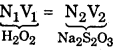N1 × 25 = 0.3 × 20Strength of H2O2 = 0.24 × 17 = 4.08 g/L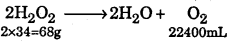68 g of H2O2 produce at NTP = 22400mL
4.08 g of H2O2 will produce O2 at NTP = $$\frac { 22400 }{ 68 }$$ × 4.08 = 1344 mL
Now 4.08 g of H2O2 are present in 1000 mL of solution.
1000 mL of H2O2 solution 1344 mL of O2 at NTP
1 mL of H2O2 solution will give = $$\frac { 1344 }{ 1000 }$$ = 1.344 mL of O2 of NTP.

Question 9.
Calculate the volume of 10 volume H2O2 required to neutralize 200 mL of 2 N KMn04 in acidic medium.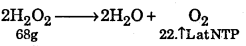22.4 L of O2 at NTP is produced from H2O2 = 68g
10L of O2 at NTP is prodced from H2O2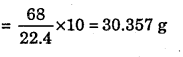Gram equivalents of H2O2 = $$\frac { 30.357 }{ 17 }$$ =1.79
Normality of H2O2 = $$\frac { 1.79 }{ 1 }$$ =1.79 N1. 79 × V1 = 2 × 200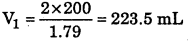Question 10.
0.5L each of three samples of H2O2 labelled 10 vol, 15 vol and 20 vol are mixed and then diluted with equal volume of water. What is the strength of resultant H2O2 solution ?
Volume strength of H2O2 solution = 5.6 × Normality
Normality = $$\frac{\text { Vol. strength }}{5.6}$$
Normality of 10 vol of H2O2 = $$\frac { 10 }{ 5.6 }$$ N
Normality of 10 vol of H2O2 = $$\frac { 15 }{ 5.6 }$$ N
Normality 20 vol of H2O2 = $$\frac { 20 }{ 5.6 }$$ N
Let 500 mL of each solution is mixed and then total volume of mixture becomes = 1500 mL. Since it is diluted with equal volume of water, total volume becomes 3000 mL.
Applying normality equation N1V2 + N2V2 + N3V3 = N4V4
$$\begin{array}{l}{\frac{10 \times 500}{5.6}+\frac{15 \times 500}{5.6}+\frac{20 \times 500}{5.6}=\mathrm{N}_{4} \times 3000} \\ {\mathrm{N}_{4}=\frac{45 \times 500}{5.6 \times 3000}=1.339 \mathrm{N}}\end{array}$$

Question 11.
Volume strength of resulting solution = 1.339 × 5.6 = 7.5 the degree of hardness of a given sample of hard water is 60 ppm. If the entire hardness is due to MgSO4, how much of MgSO4 is present per kilogram of hard water ?
Answer: Degree of hardness of water = 60ppm
Since degree of hardness is the number of parts of calcium carbonate or equivalent to calcium and magnesium salts present in a million parts of water by mass.
106 g of water contain 60 g of CaCO3
Now 1 mol of CaCCO3 = 1 mol of MgSO4
100g of CaCOa = 120g of MgSO4
106 g of water contain MgSO4 = $$\frac{60 \times 200}{100}=72 \mathrm{g}$$ = 72 g
103 g of water will contain MgSO4 = $$\frac{72}{10^{6}} \times 10^{3}$$ = 0.072 g
1 kg of water contain MgSO4 = 72 mg

Question 12.
Find the volume strength of 1.6N NH2O2 solution.
Strength = Normality × Eq. wt. Eq. wt. of H2O2 =17
∴ Strength of 1.6 NH2O2 solution = 1.6 × l7gL-1
Now 68g of H2O2 gives 22400 mL O2 atNTP/STP
∴ 1.6 × 17g of H2O2 will give = $$\frac { 22400 }{ 68 }$$ × l.6 × l7 = 8960 mL of O2 at STP
But 1.6 × 17g of H2O2 are present in 1000 mL of H2O2 solution.
Hence 1000 mL of H2O2 solution gives 8960 mL of O2 at STP
1 mL of H2O2 will give = 8.96 mL of O2 at STP
Hence the volume strength of 1.6 N H2O2 solution is = 8.96 volume.Question 13.
Calculate the volume of 10 volume H2O2 solution that will react with 200 mL of 2N KMnO4 in acidic medium.
Normality 10 volume H2O2 = $$\frac{10 \times 68}{22.4 \times 17}=\frac{10}{5.6} \mathrm{N}$$
Applying normality equation
\begin{aligned} \mathrm{N}_{1} \mathrm{V}_{1} &=\mathrm{N}_{2} \mathrm{V}_{2} \\\left(\mathrm{H}_{2} \mathrm{O}_{2}\right) &\left(\mathrm{KMnO}_{4}\right) \\ \frac{10}{5.6} \times \mathrm{V}_{1} &=2 \times 200 \\ \mathrm{V}_{1} &=\frac{2 \times 200 \times 5.6}{10}=224 \mathrm{cm}^{3} \end{aligned}

Question 14.
Calculate the percentage strength and strength in grams per litre of 10 volume hydrogen peroxide solution.
Hydrogen peroxide decomposes on heating according to the equation :From the equation,
22.4 litres of O2 at N.T.P. are obtained from 2 × 34 or 68 g of H2O2
∴ 10 ml of O2 at N.T.P. will be obtained from $$\frac { 68 }{ 22400 }$$ × 10g of H2O2
But 10 ml of O2 at N.T.P. are produced from 1 ml of 10 volume H2O2 solution
Thus 1 ml of 10 volume H2O2 solutioin contains $$\frac { 68 }{ 22400 }$$ × 10g of H2O2
∴ 100 ml of 10 volume H2O2 solution will contain $$\frac { 68 }{ 22400 }$$ × $$\frac { 10 }{ 1 }$$ x 100 = 3.036g
Thus, a 10 volume H2O2 solution is approx. 3% alternatively, 1000 ml of 10 volume
H2O2 will contain $$\frac { 68 }{ 22400 }$$ × 10 × 1000 = 30.36g
Therefore, strength of H2O2 in 10 volume
H2O2 = 30.36gL-1

Question 15.
Calculate the normality of 20 volume hydrogen peroxide solution.
To calculate the strength in g/1 of 20 volume H2O2 solution
By definition, 1 litre of 20 volume H2O2 solution on decomposition gives 20 litres of oxygen at NTP
Consider the chemical equation,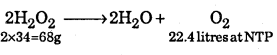Now 22.4 litres of O2 at NTP will be obtained from H2O2 = 68gThus, the strength of 20 volume H2O2 solution = 60.7 g/1

Step 2 : To calculate equivalent weight of H2O2From the above equation, 32 parts by wt. of oxygen are obtained from 68 parts by wt. of H2O2
8 parts by wt of oxygen will be obtained from $$\frac { 68 }{ 32 }$$ x 8 = 17 parts by wt. of H2O2
Eq. wt of H2O2 = 17

Step 3: To calculate the normality of 20 volume H2O2 solution
Now we know that, Normality = $$=\frac{\text { Strength }}{\text { Eq.wt }}=\frac{60.7}{17}=3.57$$
Hence the normality of 20 volume H2O2 solution = 3.57N

Question 16.
Find the volume strength of 1.6 N H2O2 solution.
We know that strength = Normality × Eq. wt; and Eq. wt of H2O2 = 17
Strength of 1.6 N H2O2 solution = 16 × 17 g/1
Now 68 g of H2O2 gives 22400 ml O2 at NTP
16 × 17 g of H2O2 will give $$\frac { 22400 }{ 68}$$ × 1.6 × 17 = 8960 ml of O2 at NTP
But 1.6 × 17 g of H2O2 are present in 1000 ml of H2O2 solution.
Hence 1000 ml of H2O2 solution gives 8960 ml of O2 at STP
lml of H2O2 solution will give = $$\frac { 8960 }{ 1000}$$ = 8.96 ml of O2 at NTP
Hence the volume strength of 1.6N H2O2 solution = 8.96 volume

Question 17.
Calculate the volume strength of a 35% solution of H2O2.
Step 1: To calculate the amount of H2O2 present in one litre of 3% solution.
100 ml of H2O2 solution contain H2O2 = 3g
1000 ml of H2O2 solution will contain H2O2 = $$\frac { 3 }{ 100}$$ x 1000 = 30 g

Step 2 : Calculate the volume strength. Consider the chemical equation.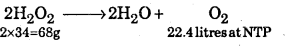Now 68 g of H2O2 give O2 at NTP = 22.4 litres
30g of H2O2 will give O2 at NTP = $$\frac { 22.4 }{ 68}$$ × 30 = 9.88 litres = 9880 ml
But 30g of H2O2 are present in 1000 ml of H2O2
Hence 1000 ml of H2O2 solution gives O2 at NTP = 9880 ml
1 ml of H2O2 solution will give O2 at NTP = $$\frac { 9880 }{ 1000}$$ = 9.88 ml
Hence the volume strength of 3% H2O2 solution = 9.88

Question 18.
What is the mass of hydrogen peroxide present in 1 litre of 2M solution ? Calculate the volume of oxygen at STP liberated upon complete decomposition of 100 cm3 of the above solution.
Step 1: To calculate the mass of H2O2 in 1 litre of 2M solution.
Molecular mass of H2O2 = 2 × 1 + 2 × 16 = 34 amu
By definition, I litre of 1M H2O2 contains 34 g of H2O2
1 litre of 2M H2O2 will contain H2O2 = 34 × 2 = 68 g

Step 2 : To calculate the volume of O2 liberated at STP fromlOO cm3 of 2M solution.
1 litre 2M H2O2 solution contains H2O2 = 68 g
100 cm3 of 2M H2O2 solution will contain $$\frac { 68 }{ 1000}$$ × 100 = 6.8g of H2O2
The equation representing the decomposition of H2O2 is
2H22O 2 × 34 = 68g → 2H2O + O22 2400cm3 at STP
Now 68 g of H2O2 at STP give O2 = 22400 cm3 k of O2
6.8 g of H2O2 at STP will evolve O2 = $$\frac { 22400 }{ 68}$$ x 6.8 = 2240cm3 = 2.24 mL regQuestion 19.
30 ml of a H2O2 solution after acidication required 30 ml of N/10 KMnCh solution for complete oxidation. Calculate the percentage and volume strength of H2O2 solution.
Step 1: To determine the normality of H2O2 solution. From the given solution.
For H2O2 V1 = 30ml, N1 = ? For KMnCh, V2 = 30ml, N2 = N/10
Applying normality equation N1V1 = N2V2
i.e., 30 × N1 = 30 × 1/10
Thus, the normality of H2O2 solution = 0.1N

Step 2: To determine the percentage strength of H2O2 solution.
We know that, H2O2 → 2H+ + O2 + 2e-
Eq. wt. of H2O2 = 30 / 2 = 17
Hence strength of H2O2 solution = Normality × Eq. wt = 0.1 × 17 = 1.7 g/litre
Percentage strength of H2O2 = $$\frac{1.7 \times 100}{1000}=0.17 \%$$

Step 3 : To determine the volume strength of H2O2 solution.
Consider the chemical equation 2H2O268g → 2H2O + O222400ml atNTP
Now 68 g of H2O2 give O2 at NTP = 22400 ml
1.7g of H2O2 will give O2 = $$\frac{22400}{68} \times 1.7=560 \mathrm{ml}$$
But 1.7 g of H2O2 are present in 1000 ml of H2O2 solution.
Hence 1000 ml of H2O2 solution gives 560 ml of O2 at NTP
1ml of H2O2 solution gives = $$\frac { 560 }{ 1000 }$$ = 0.56 ml of O2at NTP
Volume strength of H2O2 solution = 0.56

Question 20.
One litre of a sample of hard water contains Img of CaCl2 and 1 mg of MgCl2 Find out the total hardness in terms of parts of CaCO3 per 106 parts of water by mass, (i) Mol. Mass of CaCl2 = 111
Now lllg CaCl2 = lOOg of CaCO3
1 mg of CaCl2 = $$\frac { 100 }{ 111 }$$ × 1 mg of CaCO3= 0.9 mg of CaCO3

(ii) Mol. Mass of MgCk = 95
Now 95g of MgCl2 = lOOg of CaCO3
1 mg of MgCl2 = $$\frac { 100 }{ 95 }$$ × 1 mg of CaCO3 = 1.05 mg of CaCO3
Thus, 1 litre of hard water contain = 0.90 + 1.05 = 1.95 mg of CaCO3.

Question 21.
A 5.0 cm3 solution of H2O2 liberates 0.50 g of iodine from an acidified K1 solution. Calculate the strength of H2O2 solution in terms of volume strength at STP.
(a) 2K1 + H2SO4 + H2O → K2SO4 + 2H2O +I2
From the above equation, H2O2 s I2 (both are required)
34g of H2O2 = 254g of I2
∴ 0.508 g of I2 will be liberated from H2O2 = $$\frac { 34 }{ 254 }$$ x 0.508 = 0.068g

(b) The decomposition of H202 occurs as
2H2O22X34 = 68g → 2H2O + O222400 cm3 at NTP
∴ 0.068 of H2O upon decomposition will give O2 = $$\frac { 22400 }{ 68 }$$ x 0.068 = 22.4ml

(c) Now 5.0 cm3 of H2O2 solution gives O2 = 22.4cm3 at NTP
∴ 1.0 cm3 of H2O2 solution gives O2 = $$\frac { 22.4 }{ 5 }$$ = 4.48 cm3 at NTP
Thus volume strength of give H2O2 solution = 4.48

Question 22.
Calculate molarity and normality of 20 volume of H2O2. What is its mass percentage?
vol. of H2O2 means 1 ml of H2O2 gives 20ml of O2 at STP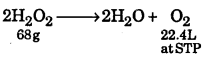22400 ml of O2 of STP is given by 68g of H2O2
20 ml of O2 at STP is given by $$\frac { 68 }{ 22400 }$$ x 20g of H2 = 0.0606 H2O2
1000 ml of H2O2 contains 0.0606 × 1000 = 60.6 = 60.6
∴ Molarity = $$\frac{60.6}{\text { Mol. wt of } \mathrm{H}_{2} \mathrm{O}_{2}}$$
∴ M = $$\frac { 60.6 }{ 34.0 }$$ = 1.78 $$\left[\because \mathrm{Eqwt}=\frac{\mathrm{mol} \mathrm{wt}}{2}\right]$$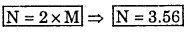Question 23.
20 ml of solution having 0.2 g of impure H2O2 reacts with 0.316 of KMnO4 in acidic medium. Calculate (a) Purity of H2O2 (b) Volume of dry oxygen evolved at 0° C and 750 mm pressure.
0.316 g of KMnO4 will react with $$\frac { 170 }{ 0.2 }$$ x 0.316 g of H2O2 = 0.169 g of H2O2
=> Percentage purity of H2O2 = $$\frac { 0.169 }{ 0.2 }$$ x 100 = 85%